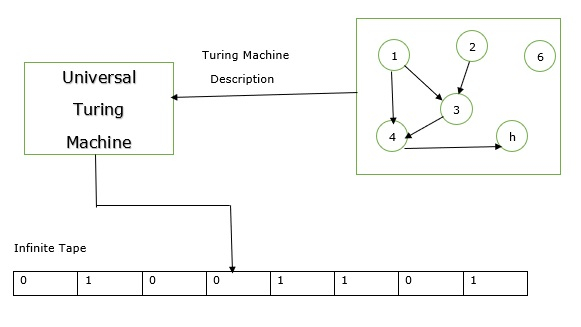# Explain the universal Turing machine in TOC

The Turing Machine (TM) is the machine level equivalent to a digital computer.

It was suggested by the mathematician Turing in the year 1930 and has become the most widely used model of computation in computability and complexity theory.

The model consists of an input and output. The input is given in binary format form on to the machine’s tape and the output consists of the contents of the tape when the machine halts

The problem with the Turing machine is that a different machine must be constructed for every new computation to be performed for every input output relation.

This is the reason the Universal Turing machine was introduced which along with input on the tape takes the description of a machine M.

The Universal Turing machine can go on then to simulate M on the rest of the content of the input tape.

A Universal Turing machine can thus simulate any other machine.

The idea of connecting multiple Turing machine gave an idea to Turing −

• Can a Universal machine be created that can ‘simulate’ other machines?

• This machine is called as Universal Turing Machine

This machine would have three bits of information for the machine it is simulating

• A basic description of the machine.
• The contents of machine tape.
• The internal state of the machine.

The Universal machine would simulate the machine by looking at the input on the tape and the state of the machine.

It would control the machine by changing its state based on the input. This leads to the idea of a “computer running another computer”.

It would control the machine by changing its state based on the input. This leads to the idea of a “computer running another computer”.

The schematic diagram of the Universal Turing Machine is as follows −